# Have solution

The sum of four consecutive even numbers is 92. Determine these numbers.

a =  20
b =  22
c =  24
d =  26

### Step-by-step explanation:

a+b+c+d=92
b=a+2
c=b+2
d=c+2

a+b+c+d = 92
a-b = -2
b-c = -2
c-d = -2

a = 20
b = 22
c = 24
d = 26

Our linear equations calculator calculates it.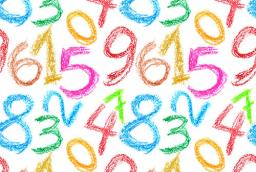Did you find an error or inaccuracy? Feel free to write us. Thank you!Tips to related online calculators
Do you have a linear equation or system of equations and looking for its solution? Or do you have a quadratic equation?

## Related math problems and questions:

• Odd numbersThe sum of four consecutive odd numbers is 1048. Find those numbers ...
• Unknown numbersThe sum of two consecutive natural numbers and their triple is 92. Find these numbers.
• The sumThe sum of five consecutive odd numbers is 75. Find out the sum of the second and fourth of them.
• Consecutive numbersSum of ten consecutive numbers is 105. Determine these numbers (write first and last).
• MartinaMartina is solving the equation 4x - 11 = 2x + 391. Here are the first steps of her solution. 4x - 11 = 2x + 391 2x - 11 = 391 2x = 402 What did Martina do to get 2x - 11 = 391?
• Sum of four numbers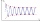The sum of four consecutive natural numbers is 114. Find them.
• Three ints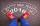The sum of three consecutive integers is 2016. What numbers are they?
• AM of three numbersThe number 2010 can be written as the sum of 3 consecutive natural numbers. Determine the arithmetic mean of these numbers.
• The sum 2The sum of five consecutive even integers is 150. Find the largest of the five integers. A.28 B.30 C.34 D.54 Show your solution and explain your answer.
• Sum of three numbersThe sum of three numbers from which the second number is 20% smaller than the first number and the third number is 25% smaller than the second number is 96. Determine these numbers.
• Real estate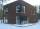The residential house has three entrances numbered by odd numbers arithmetic progression. The sum of the two numbers on the corner entrances is 50. Calculate the highest of these three numbers.
• How manyHow many numbers are less than 222 with a digit sum is 8?
• HarryHarry Thomson bought a large land in the shape of a rectangle with a circumference of 90 meters. He divided it into three rectangular plots. The shorter side has all three plots of equal length, their longer sides are three consecutive natural numbers. Fi
• Ratio three numbers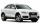Three numbers SUV are in the ratio 1:2:3. Their sum is 24. Find this numbers and write their add and sum.
• Arithmetic progression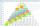In some AP applies: 5a2 + 7a5 = 90 s3 = 12 Find the first member a =? and difference d = ?
• Last pageTwo consecutive sheets dropped out of the book. The sum of the numbers on the sides of the dropped sheets is 154. What is the number of the last page of the dropped sheets?
• Even / odd numbers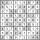a / Using variable n write two consecutive odd numbers. b / the sum of three consecutive odd numbers is 333. What are this numbers?